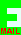## PRIZE PUZZLE FOR SEPTEMBER 2004The "Ladders Against The Wall" problem shown here is well known. I first came across this problem when studying mathematics as a young man. I spent hours trying to solve the equation for x and eventually gave up. Coming back to it many years later I resolved it into a fourth order polynomial in x2 which is still difficult (but not impossible) to work out analytically. The generally best way to solve it is using a numerical method with the help of a computer program.

But this month I have chosen a special case. I believe that this is the smallest version of the problem that has an integer answer with integer values for the ladders and the height of the crossover point. This should make it easier because a few inspired guesses will resolve the problem.

Please send me the value of x by 6th October to be entered into the prize draw. If you can find values of the three parameters that sum to a smaller value than 219 and still give an integer answer you will get a special mention! Best of luck. PS: The ladders should be of different lengths.

To contact me by email click this symbol:SOLUTION

The answer is 56. John Stafford showed this to be the smallest puzzle of this type in integers.

I received two correct answers: one from John Stafford and one from Clem Robertson, but there was no draw this month as there were only two competitors (a recent new rule requires that at least three competitors must take part in the draw).

Given that the result was a whole number, you could make a rough scale drawing and measure the width, round to the nearest integer and check the answer using pythagoras.

Another method is to just make some guesses and test to see if they fit the geometry of the diagram.

The mathematical method for non-integer cases involves finding the solution to a fourth order polynomial equation. I would not expect anyone to attempt this, but numerical methods exist for solving equations that could be employed. I have even implemented such a scheme on Microsoft's Works Spreadsheet that gives an accurate answer to 14 decimal digits.

[home] [back]